Published

# 8 Watering Incidence, 16 Zone Sprinkler System Circuit

This is a custom garden watering system replacement circuitry for multiple sprinkler boxes, phase 1, with flow meter addition.

IntermediateWork in progress4 hours748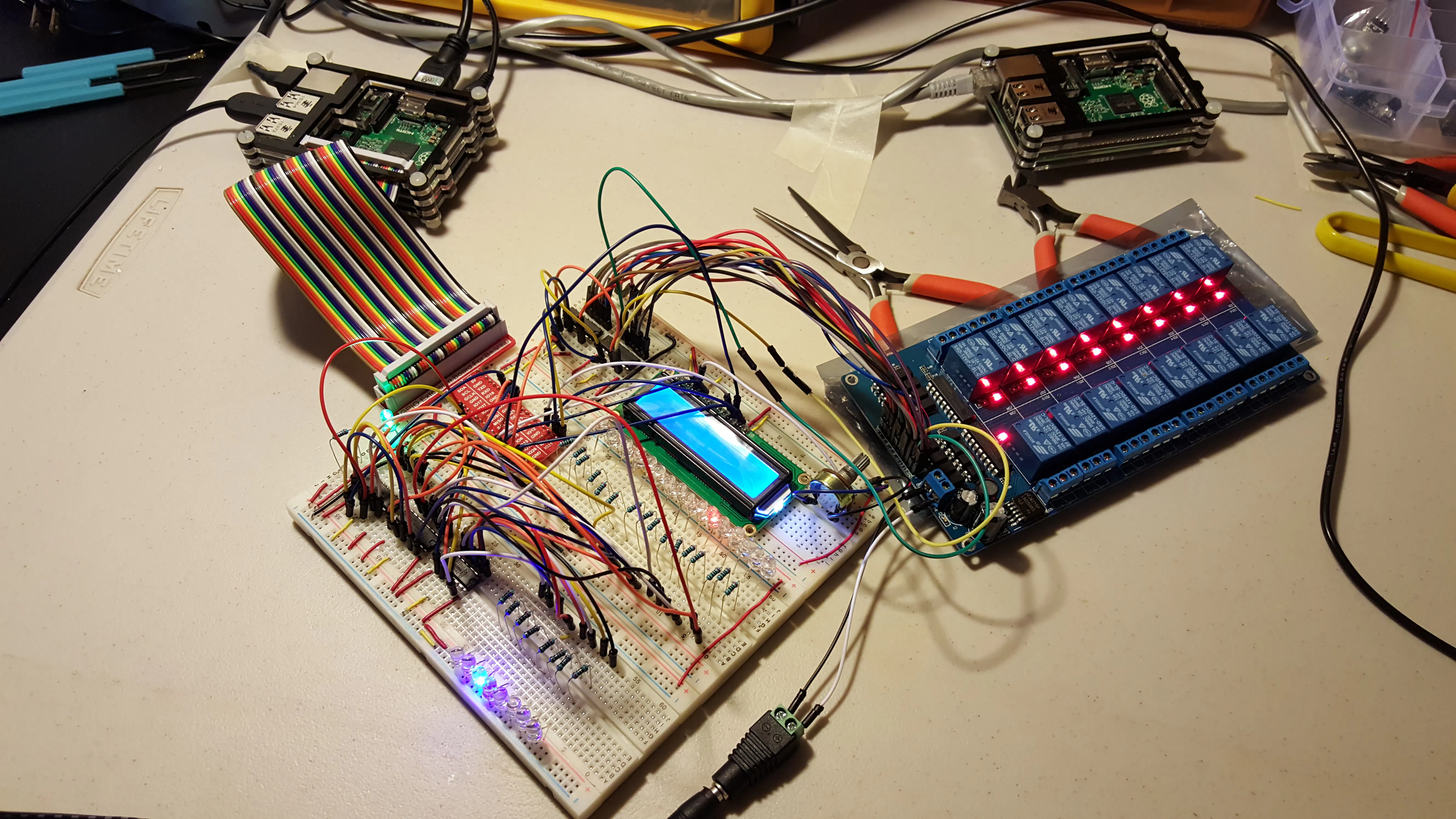## Things used in this project

### Hardware components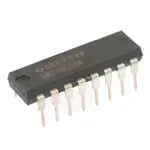Texas Instruments Shift Register- Serial to Parallel
×5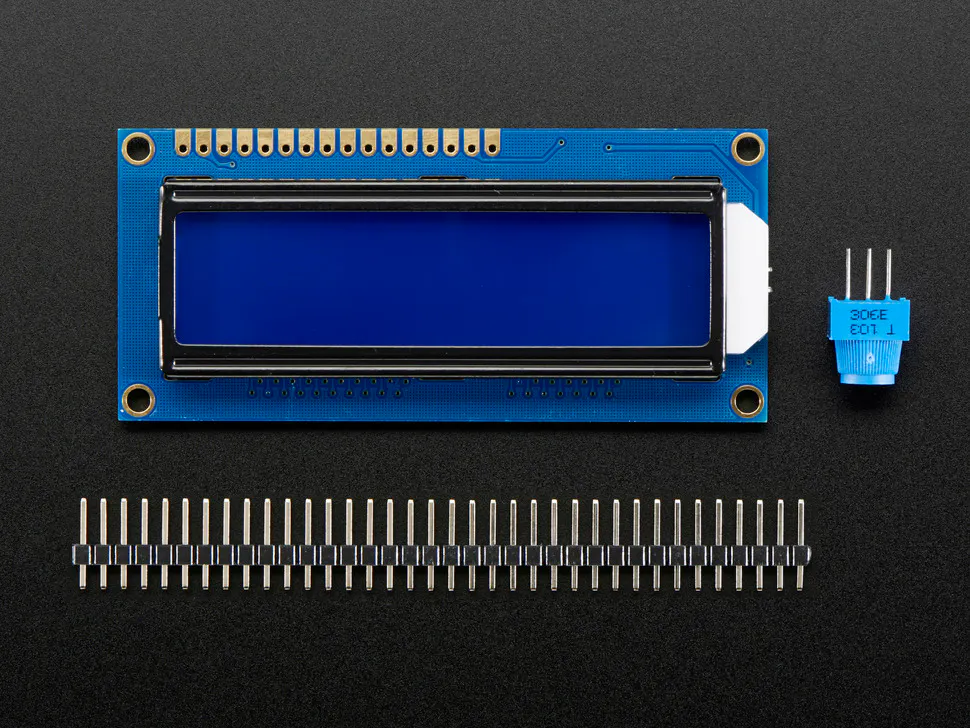Adafruit Standard LCD - 16x2 White on Blue
×1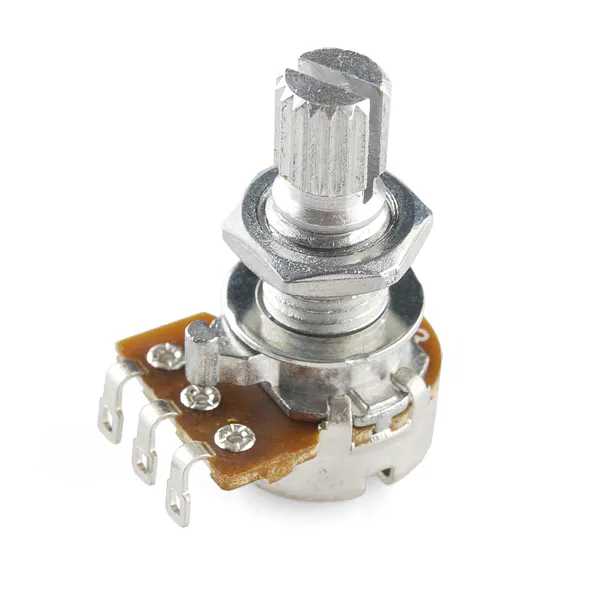Rotary potentiometer (generic)
×1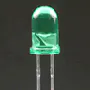5 mm LED: Green
×15 mm LED: Red
×16
 5 mm LED Blue
×8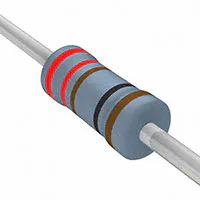Resistor 221 ohm
×25
 flow sensor
×1

## Schematics

### 8 Run Incidence 16 Zone Sprinkler Fritz

Phase 1 of a multi phase garden watering and monitoring system.

## Code

### sprinkler system base code

Python
base code that when run looks for a input file to tell it what incidence this is and how long each zone should run. A lot of this code should look familiar as variations on a theme in what you find in Sunfounder, Adafruit, etc.
```#!/usr/bin/env python
import RPi.GPIO as GPIO
import time
import sys
import os

#Flow Sensor
FLOW_SENSOR = 31

calibrationFactor = 4.8
global count
count = 0
global maxcount
maxcount = 0

# LCD PIN OUT
# The wiring for the LCD is as follows:
# 1 : GND
# 2 : 5V
# 3 : Contrast (0-5V)*
# 4 : RS (Register Select)
# 5 : R/W (Read Write)       - GROUND THIS PIN
# 6 : Enable or Strobe
# 7 : Data Bit 0             - NOT USED
# 8 : Data Bit 1             - NOT USED
# 9 : Data Bit 2             - NOT USED
# 10: Data Bit 3             - NOT USED
# 11: Data Bit 4
# 12: Data Bit 5
# 13: Data Bit 6
# 14: Data Bit 7
# 15: LCD Backlight +5V**
# 16: LCD Backlight GND

#LCD SETUP 4 bit mode
# Define GPIO to LCD mapping
LCD_RS = 33
LCD_E  = 35
LCD_D4 = 36
LCD_D5 = 37
LCD_D6 = 38
LCD_D7 = 40
LED_ON = 32
# Define some device constants
LCD_WIDTH = 16    # Maximum characters per line
LCD_CHR = True
LCD_CMD = False
LCD_LINE_1 = 0x80 # LCD RAM address for the 1st line
LCD_LINE_2 = 0xC0 # LCD RAM address for the 2nd line
# Timing constants
E_PULSE = 0.00005
E_DELAY = 0.00005

# session LED Chip (8 sessions)
SDI3 = 15
RCLK3 = 16
SRCLK3 = 18
# map to chip outputs
LedGroup1 = [0x01,0x02,0x04,0x08,0x10,0x20,0x40,0x80]

# zone LED chips (16 zones)
SDI   = 11
RCLK  = 12
SRCLK = 13
# relay chips
SDI2   = 19
RCLK2  = 21
SRCLK2 = 22

# map to chip outputs
WhichLeds = [0x01,0x02,0x04,0x08,0x10,0x20,0x40,0x80,0x100,0x200,0x400,0x800,0x1000,0x2000,0x4000,0x8000]

def setup():
# LED's (incidence and zone)
GPIO.setmode(GPIO.BOARD)	# Number GPIOs by its physical location
GPIO.setup(FLOW_SENSOR, GPIO.IN, pull_up_down=GPIO.PUD_UP)
GPIO.setup(SDI, GPIO.OUT)	# input for zone LED's  1-16
GPIO.setup(SDI2, GPIO.OUT)	# input for Relay's 1-16
GPIO.setup(SDI3, GPIO.OUT)	# input for watering incidence 1-8
GPIO.setup(RCLK, GPIO.OUT)
GPIO.setup(RCLK2, GPIO.OUT)
GPIO.setup(RCLK3, GPIO.OUT)
GPIO.setup(SRCLK, GPIO.OUT)
GPIO.setup(SRCLK2, GPIO.OUT)
GPIO.setup(SRCLK3, GPIO.OUT)
GPIO.setup(LCD_E, GPIO.OUT)  # E
GPIO.setup(LCD_RS, GPIO.OUT) # RS
GPIO.setup(LCD_D4, GPIO.OUT) # DB4
GPIO.setup(LCD_D5, GPIO.OUT) # DB5
GPIO.setup(LCD_D6, GPIO.OUT) # DB6
GPIO.setup(LCD_D7, GPIO.OUT) # DB7
GPIO.setup(LED_ON, GPIO.OUT) # Backlight enable

# LCD inputs
# set initial States
GPIO.output(SDI, GPIO.LOW)
GPIO.output(RCLK, GPIO.LOW)
GPIO.output(SRCLK, GPIO.LOW)
GPIO.output(SDI2, GPIO.LOW)
GPIO.output(RCLK2, GPIO.LOW)
GPIO.output(SRCLK2, GPIO.LOW)
GPIO.output(SDI3, GPIO.LOW)
GPIO.output(RCLK3, GPIO.LOW)
GPIO.output(SRCLK3, GPIO.LOW)
# Initialise display
lcd_byte(0x33,LCD_CMD)
lcd_byte(0x32,LCD_CMD)
lcd_byte(0x28,LCD_CMD)
lcd_byte(0x0C,LCD_CMD)
lcd_byte(0x06,LCD_CMD)
lcd_byte(0x01,LCD_CMD)

def countPulseReset():
global count
count = 0

def countPulse(channel):
global count
count = count + 1

def lcd_string(message,style):
# Send string to display
# style=1 Left justified
# style=2 Centred
# style=3 Right justified
if style==1:
message = message.ljust(LCD_WIDTH," ")
elif style==2:
message = message.center(LCD_WIDTH," ")
elif style==3:
message = message.rjust(LCD_WIDTH," ")

for i in range(LCD_WIDTH):
lcd_byte(ord(message[i]),LCD_CHR)

def lcd_byte(bits, mode):
# Send byte to data pins
# bits = data
# mode = True  for character
#        False for command

GPIO.output(LCD_RS, mode) # RS
# High bits
GPIO.output(LCD_D4, False)
GPIO.output(LCD_D5, False)
GPIO.output(LCD_D6, False)
GPIO.output(LCD_D7, False)
if bits&0x10==0x10:
GPIO.output(LCD_D4, True)
if bits&0x20==0x20:
GPIO.output(LCD_D5, True)
if bits&0x40==0x40:
GPIO.output(LCD_D6, True)
if bits&0x80==0x80:
GPIO.output(LCD_D7, True)
# Toggle 'Enable' pin
time.sleep(E_DELAY)
GPIO.output(LCD_E, True)
time.sleep(E_PULSE)
GPIO.output(LCD_E, False)
time.sleep(E_DELAY)
# Low bits
GPIO.output(LCD_D4, False)
GPIO.output(LCD_D5, False)
GPIO.output(LCD_D6, False)
GPIO.output(LCD_D7, False)
if bits&0x01==0x01:
GPIO.output(LCD_D4, True)
if bits&0x02==0x02:
GPIO.output(LCD_D5, True)
if bits&0x04==0x04:
GPIO.output(LCD_D6, True)
if bits&0x08==0x08:
GPIO.output(LCD_D7, True)
# Toggle 'Enable' pin
time.sleep(E_DELAY)
GPIO.output(LCD_E, True)
time.sleep(E_PULSE)
GPIO.output(LCD_E, False)
time.sleep(E_DELAY)

def zones_in(dat):
#	print dat
for bit in range(0, 16):
GPIO.output(SDI, 0x8000 & (dat << bit))
GPIO.output(SDI2, 0x8000 & (dat << bit))
GPIO.output(SRCLK, GPIO.HIGH)
GPIO.output(SRCLK2, GPIO.HIGH)
time.sleep(0.01)
GPIO.output(SRCLK, GPIO.LOW)
GPIO.output(SRCLK2, GPIO.LOW)

def zones_out():
GPIO.output(RCLK, GPIO.HIGH)
GPIO.output(RCLK2, GPIO.HIGH)
time.sleep(0.01)
GPIO.output(RCLK, GPIO.LOW)
GPIO.output(RCLK2, GPIO.LOW)

def zones_clear():
for bit in range (0,16):
GPIO.output(SDI, 0x8000 & ( 0 << bit))
GPIO.output(SDI2, 0x8000 & ( 0 << bit))
GPIO.output(SRCLK, GPIO.HIGH)
GPIO.output(SRCLK2, GPIO.HIGH)
time.sleep(0.01)
GPIO.output(SRCLK, GPIO.LOW)
GPIO.output(SRCLK2, GPIO.LOW)

def runs_in(dat):
#	print "DAT: " + str(dat)
for bit in range(0, 8):
GPIO.output(SDI3, 0x80 & (dat << bit))
GPIO.output(SRCLK3, GPIO.HIGH)
time.sleep(0.01)
GPIO.output(SRCLK3, GPIO.LOW)

def runs_out():
GPIO.output(RCLK3, GPIO.HIGH)
time.sleep(0.1)
GPIO.output(RCLK3, GPIO.LOW)

def runs_clear():
GPIO.output(SDI3, GPIO.LOW)
for bit in range(0,8):
GPIO.output(SDI3, 0x80 & ( 0 << bit))
GPIO.output(SRCLK3, GPIO.HIGH)
time.sleep(0.01)
GPIO.output(SRCLK3, GPIO.LOW)

def doRun():
INCIDENCE = ""
#	setRunVariables()
#
f = open("/home/pi/doRun.txt")
f.close()
print runInfo
runArray = runInfo.split(" ")
print "runArray: " + runArray
INCIDENCE = runArray
print "INCIDENCE: " + INCIDENCE
try:
ZONE1Time = runArray
except:
ZONE1Time = 0
try:
ZONE2Time = runArray
except:
ZONE2Time = 0
try:
ZONE3Time = runArray
except:
ZONE3Time = 0
try:
ZONE4Time = runArray
except:
ZONE4Time = 0
try:
ZONE5Time = runArray
except:
ZONE5Time = 0
try:
ZONE6Time = runArray
except:
ZONE6Time = 0
try:
ZONE7Time = runArray
except:
ZONE7Time = 0
try:
ZONE8Time = runArray
except:
ZONE8Time = 0
try:
ZONE9Time = runArray
except:
ZONE9Time = 0
try:
ZONE10Time = runArray
except:
ZONE10Time = 0
try:
ZONE11Time = runArray
except:
ZONE11Time = 0
try:
ZONE12Time = runArray
except:
ZONE12Time = 0
try:
ZONE13Time = runArray
except:
ZONE13Time = 0
try:
ZONE14Time = runArray
except:
ZONE14Time = 0
try:
ZONE15Time = runArray
except:
ZONE15Time = 0
try:
ZONE16Time = runArray
except:
ZONE16Time = 0
ZoneArray = [ZONE1Time, ZONE2Time, ZONE3Time, ZONE4Time, ZONE5Time, ZONE6Time, ZONE7Time, ZONE8Time, ZONE9Time, ZONE10Time, ZONE11Time, ZONE12Time, ZONE13Time, ZONE14Time, ZONE15Time, ZONE16Time]

#print ZONE1Time
#print ZONE2Time
#print ZONE3Time
#print ZONE4Time
#print ZONE5Time
#print ZONE6Time
#print ZONE7Time
#print ZONE8Time
#print ZONE9Time
#print ZONE10Time
#print ZONE11Time
#print ZONE12Time
#print ZONE13Time
#print ZONE14Time
#print ZONE15Time
#print ZONE16Time
print "INCIDENCE RETURN: " + INCIDENCE
# Toggle backlight on-off-on
GPIO.output(LED_ON, True)
#	time.sleep(1)
#	GPIO.output(LED_ON, False)
#	time.sleep(1)
#	GPIO.output(LED_ON, True)
time.sleep(1)
lcd_byte(LCD_LINE_1, LCD_CMD)
lcd_string("",2)
lcd_byte(LCD_LINE_2, LCD_CMD)
lcd_string("",2)
# Send some centred test
lcd_byte(LCD_LINE_1, LCD_CMD)
lcd_string("Run: " + str(INCIDENCE),2)
lcd_byte(LCD_LINE_2, LCD_CMD)
lcd_string("",2)
#	time.sleep(2)
#	for i in range(0, len(LedGroup1)):
print "Run: " + INCIDENCE
print str(int(INCIDENCE))
#	runs_in(LedGroup1[i])
runs_in(LedGroup1[int(INCIDENCE)-1])
runs_out()
print "run set"
time.sleep(1)
for i in range(0, len(WhichLeds)):
if int(ZoneArray[i]) > 0:
zone = str(int(i + 1))
lcd_byte(LCD_LINE_1, LCD_CMD)
lcd_string("Run: "+ INCIDENCE + " Zone:" + zone,2)
print "Zone: " + zone + " Seconds: " +  ZoneArray[i]
zones_in(WhichLeds[i])
zones_out()
runs_clear()
runs_out()
runs_in(LedGroup1[int(INCIDENCE)-1])
runs_out()
ztime = int(ZoneArray[i])
#			print "Zone Time: " + ZoneArray[i] + " seconds"
global maxcount
maxcount = 0
for i in range(0, int(ZoneArray[i])):
lcd_byte(LCD_LINE_2, LCD_CMD)
lcd_string("Seconds: " + str(ztime),2)
#print str(ztime)
ztime = int(ztime-1)
countPulseReset()
time.sleep(1)
GPIO.remove_event_detect(FLOW_SENSOR)
if count > maxcount:
maxcount = count
#print "Count: " + str(count)
# hold position open until time length finished.
print "Zone Max Count: " + str(maxcount)
flowLperM = maxcount / calibrationFactor
flowRate = str(float("{0:.2f}".format(flowLperM)))
print "Flow L/m: " + flowRate
#			flowRate = int(ZoneArray[i])

lcd_byte(LCD_LINE_1, LCD_CMD)
lcd_string("",2)
lcd_byte(LCD_LINE_2, LCD_CMD)
lcd_string("",2)
#	time.sleep(5)
GPIO.output(LED_ON, False)

def print_msg():
print 'Program is running...'
print 'Please press Ctrl+C to end the program...'

def destroy():   # When program ending, the function is executed.
GPIO.cleanup()

if __name__ == '__main__': # Program starting from here
print_msg()
setup()
zones_clear()
zones_out()
runs_clear()
runs_out()
try:
while True:
if (os.path.isfile("/home/pi/doRun.txt")):
print "doing run"
doRun()
os.remove("/home/pi/doRun.txt")
INCIDENCE = ""
runs_clear()
runs_out()
zones_clear()
zones_out()
print "sleeping 10 seconds waiting for file"
time.sleep(10)
except KeyboardInterrupt:
destroy()
```

### example of 'doRun.txt'

Plain text
this file is what the code looks for to kick off a run.
param 0: watering run
param 1-16: how long each zone runs
```1 1200 1200 1200 600 600 600 600 600 600 600 600 600 600 600 600
```

## Credits

### Rich Noordam

10 projects • 28 followers
Many interests, computing obviously being one of them. MS SQL Server Database Administration, Reporting, Data Science, and more.